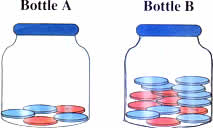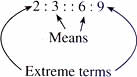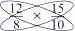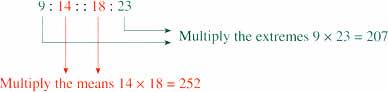Tuesday , July 5 2022# NCERT 5th Class (CBSE) Mathematics: Ratio And Proportion

## PROPORTION

Here are two bottles. Both contain red and blue counters.Bottle A has 2 red counters and 3 blue counters.

Ratio of red to blue counters is 2 : 3 in bottle A.

Bottle B has 6 red counters and 9 blue counters.

Ratio of red to blue counters is 6 : 9 in bottle B.

But, the ratios 2 : 3 and 6 : 9 are equal because 2 ( × 3 )/3 ( × 3 ) =  6/9

As the two ratios are equal we can say that are in proportion and write it as 2 : 3 : : 6 : 9

This is read as 2 is to 3 as 6 is to 9

In the proportion 2 : 3 : : 6 : 9, 2 and 9 are called the extreme terms and 3 and 6 are the mean or middle terms.(a) A recipe requires 12 tomatoes to make 8 cups of soup. Another recipe needs 15 tomatoes to make 10 cups of soup. How do we find out if the two recipes have the same proportion of tomatoes to cups of soup?

We can say that the ratio of tomatoes to the cups of soup for the first recipe is 12 : 8 or 12/8

The ratio for the second recipe is 15 : 10 or 15/10

To test whether the two ratios are proportionate we can use cross – multiplication.8 × 15 = 120 and 12 × 10 = 120

The cross products are equal; so the ratios are in proportion.

i.e., 12 : 18 : : 15 : 10

So the two recipes have the same proportion of tomatoes to cups of soup.

(b) Another way to look at this is to check whether the product of the means is equal to the product of the product of the extremes.

To check the proportionality of the ratios 9 : 14 and 18 : 23The ratios are not in proportion as the products are not equal.

### Using Proportion

(a) Aarti uses 4 eggs to make breakfast for 3 people. How many eggs would she need to make breakfast for 18 people?

The ratio of the number of eggs to the number of people is 4/3

4 eggs/3 people = ? eggs/18 people

As a proportion 4 : 3 : : ? : 18

4/3 = ?/18

4 (×6)/3 (×6) = 24/18

Answer: Aarti needs 24 eggs to make breakfast for 18 people.

(b) 4 rakhis cost Rs. 32. How much money will be need to buy 7 rakhis?

4 : 32 : : 7 : ?

4/32 = 1/8 (lowest terms)

1 : 8 : : 7 : ? or 1/8 = 7/?

1 × 7 / 8 × 7 = 7/56

Answer: 7 rakhis will coast Rs. 56.

## चुनौती हिमालय की 5th NCERT CBSE Hindi Rimjhim Ch 18

चुनौती हिमालय की 5th Class NCERT CBSE Hindi Book Rimjhim Chapter 18 प्रश्न: लद्दाख जम्मू-कश्मीर राज्य में …